Examples

Chapter 11 Class 9 Constructions [Deleted]
Serial order wise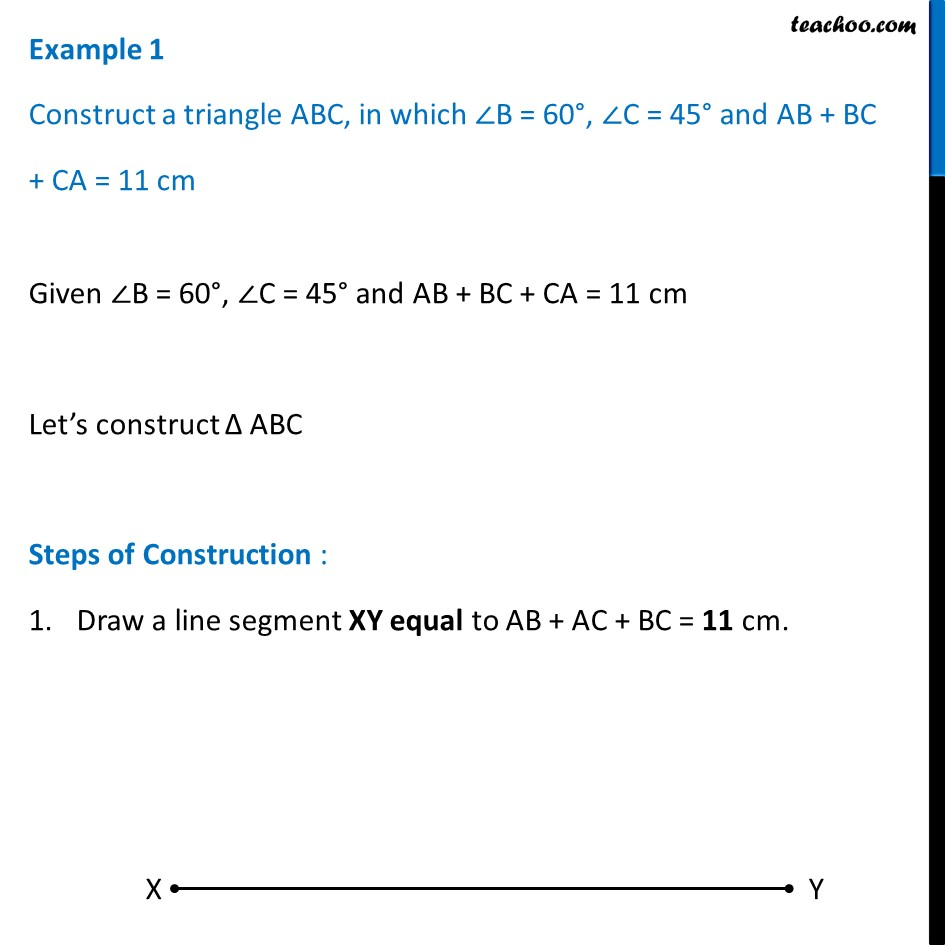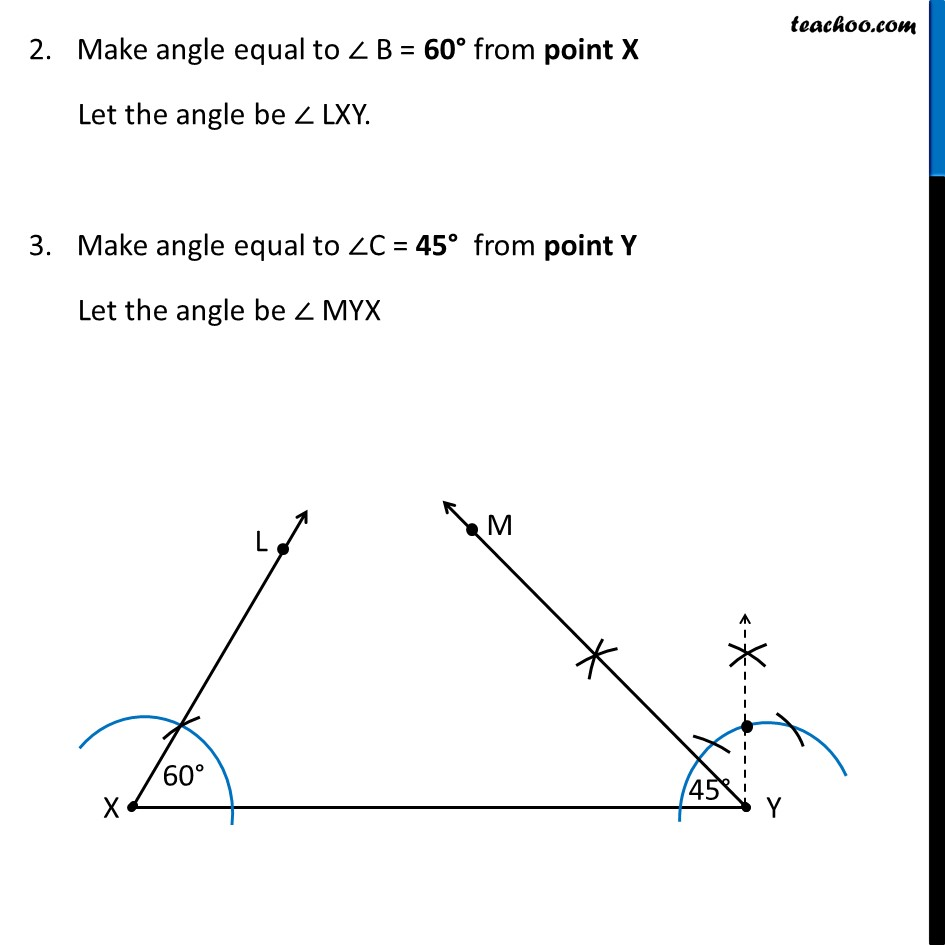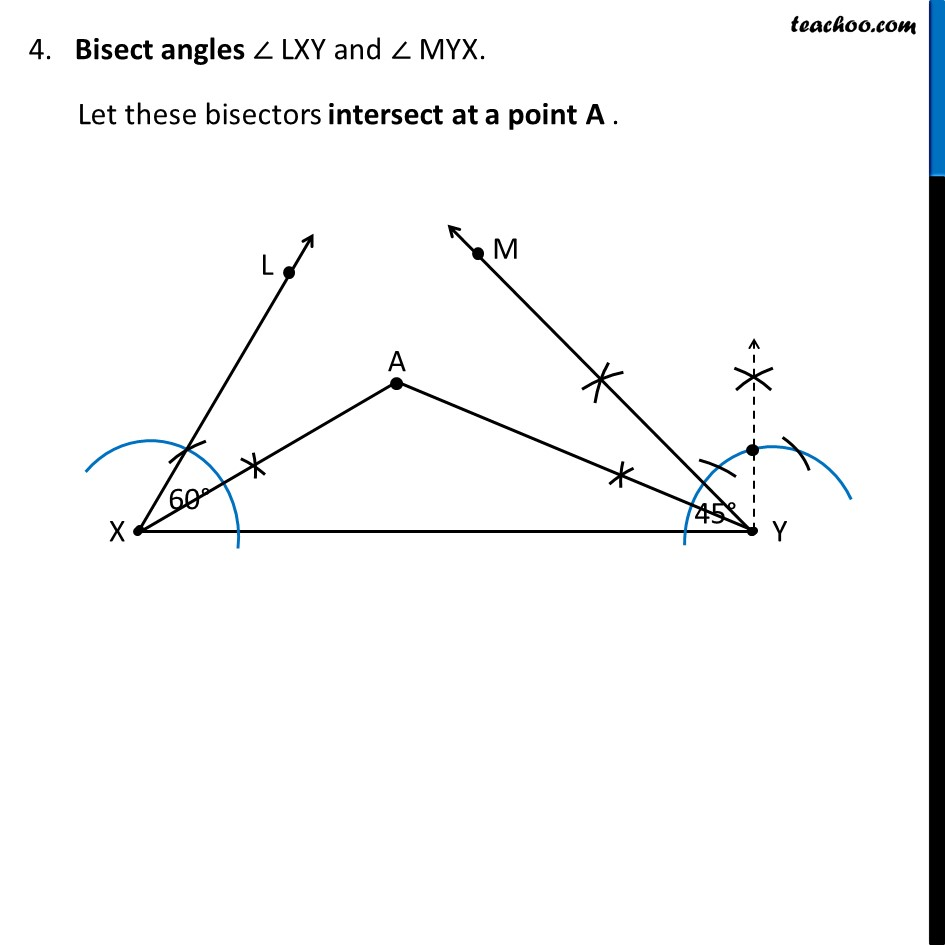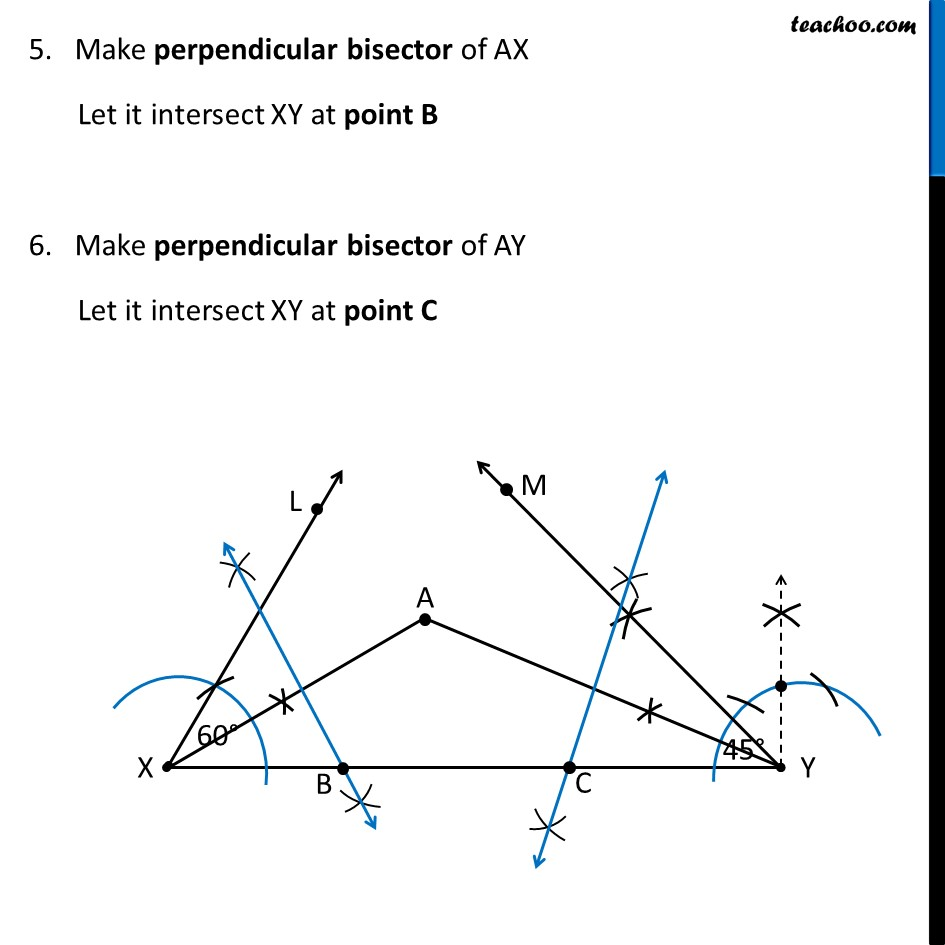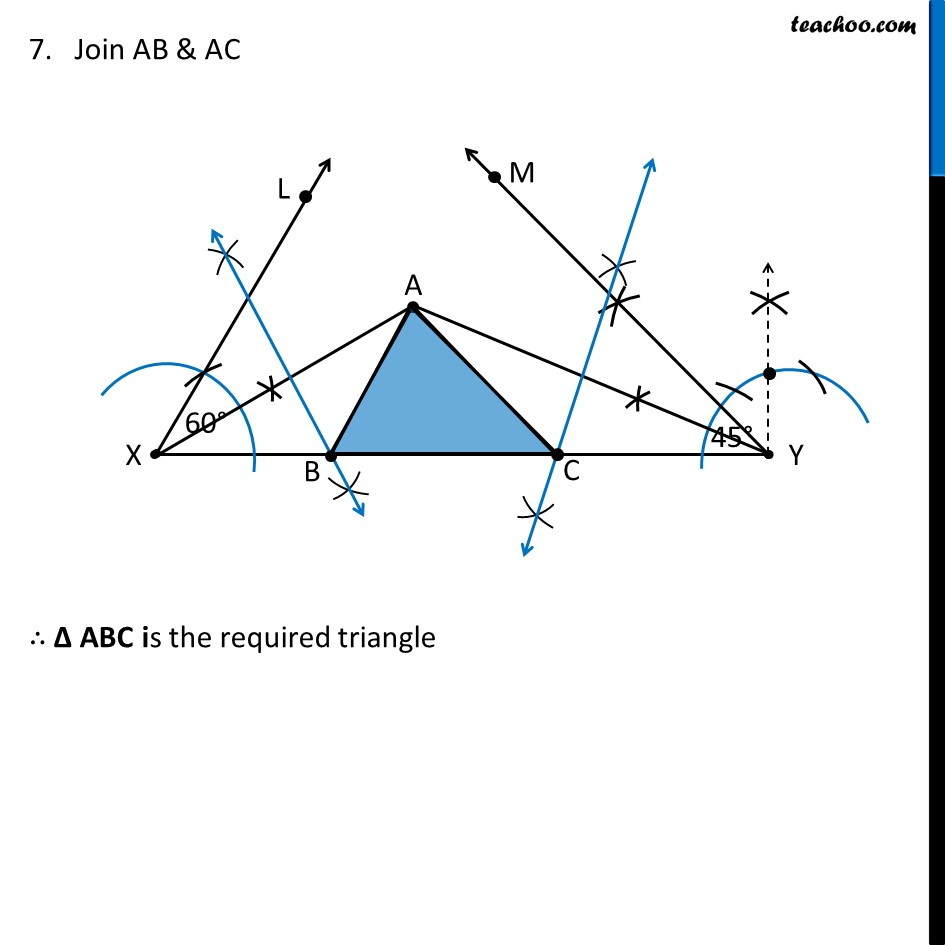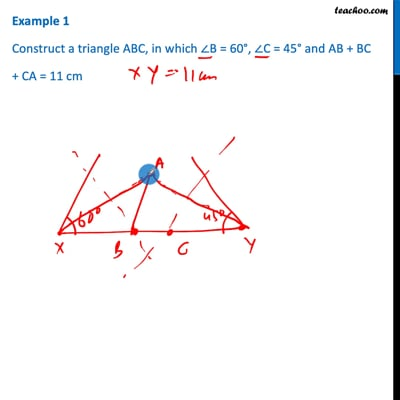This video is only available for Teachoo black users

Maths Crash Course - Live lectures + all videos + Real time Doubt solving!

### Transcript

Example 1 Construct a triangle ABC, in which ∠B = 60°, ∠C = 45° and AB + BC + CA = 11 cm Given ∠B = 60°, ∠C = 45° and AB + BC + CA = 11 cm Let’s construct Δ ABC Steps of Construction : Draw a line segment XY equal to AB + AC + BC = 11 cm. Make angle equal to ∠ B = 60° from point X Let the angle be ∠ LXY. Make angle equal to ∠C = 45° from point Y Let the angle be ∠ MYX 4. Bisect angles ∠ LXY and ∠ MYX. Let these bisectors intersect at a point A . 5. Make perpendicular bisector of AX Let it intersect XY at point B 6. Make perpendicular bisector of AY Let it intersect XY at point C 7. Join AB & AC ∴ Δ ABC is the required triangle